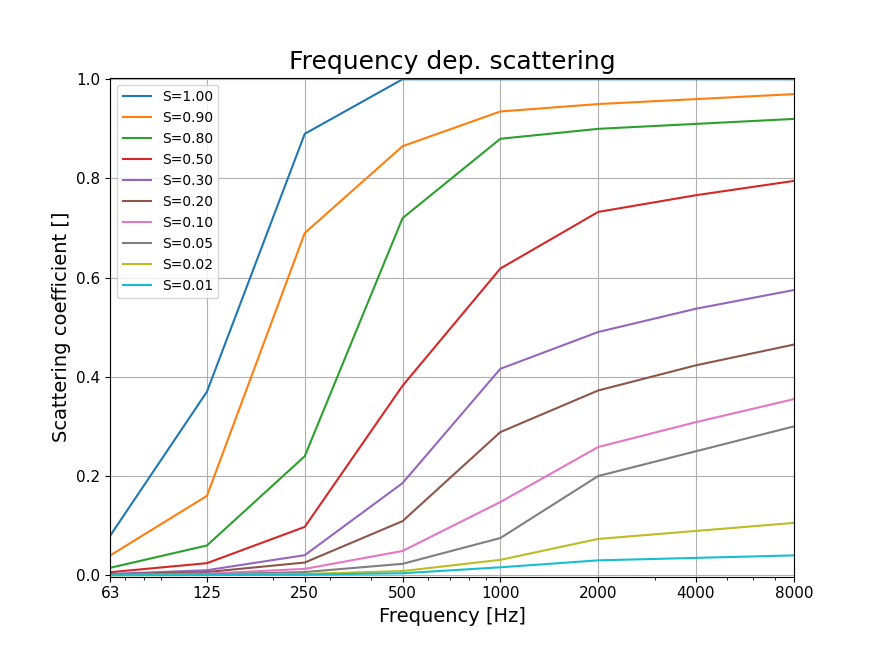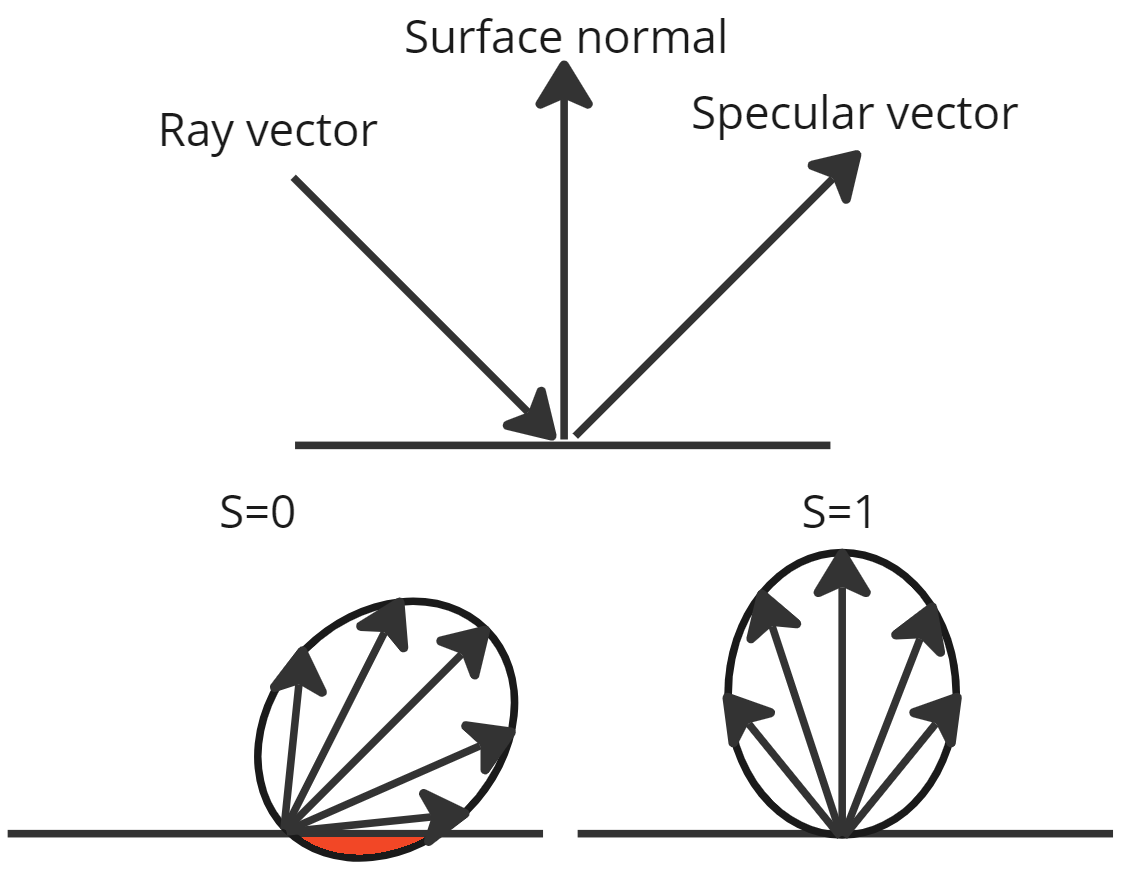# Scattering

The definition of the scattering coefficient is that it denotes the ratio of sound energy that is reflected in other directions than the specular direction. Therefore the scattering coefficient is always a value between 0 and 1, where 0 means that the reflected wave is fully specular and 1 means that it is fully diffuse. The scattering coefficient is to a large extend a concept that needs to be included into GA due to the lack of diffraction and other wave-effects in the basic underlying approximations and assumptions.

It is possible to assign a single scattering coefficient to a surface or a scattering coefficient pr octave band. These two types of inputs triggers quite different behavior in the solver and are not expected to be able to give the same results.

With both the single and the octave band input it is necessary to introduce the concept of a "Trace Scattering Coefficient". The trace coefficient is only used in the ray-radiosity solver, where it is used only when tracing the ray around the space. i.e. to calculate the new direction for the radiosity-rays.

In the case of the single value input, the trace scattering coefficient is the same for all octave bands, whereas for the octave band version the input is used directly.

For all other application of the scattering coefficients the single value scattering coefficient used to extrapolate a scattering pr octave band curve as shown in the plot below.The image source method will use the extrapolated curve or the user input octave band values when the scattered energy is subtracted. For the pressure based method, a band filtered frequency response is used for this.

The ray-radiosity solver will use this scattering coefficient in the step where the energy is traced back from the surface hitpoints to the receivers. Here the scattering coefficient is used to scale the amount of energy being registered. This works by angling the lambert distribution towards either the surface normal (scattering=1) and towards the specular vector (scattering=0). To conserve the energy, the distribution is scaled by the factor of the distribution that falls behind the surface. The figure illustrates the 2d case, but the concepts are similar in the 3d case.